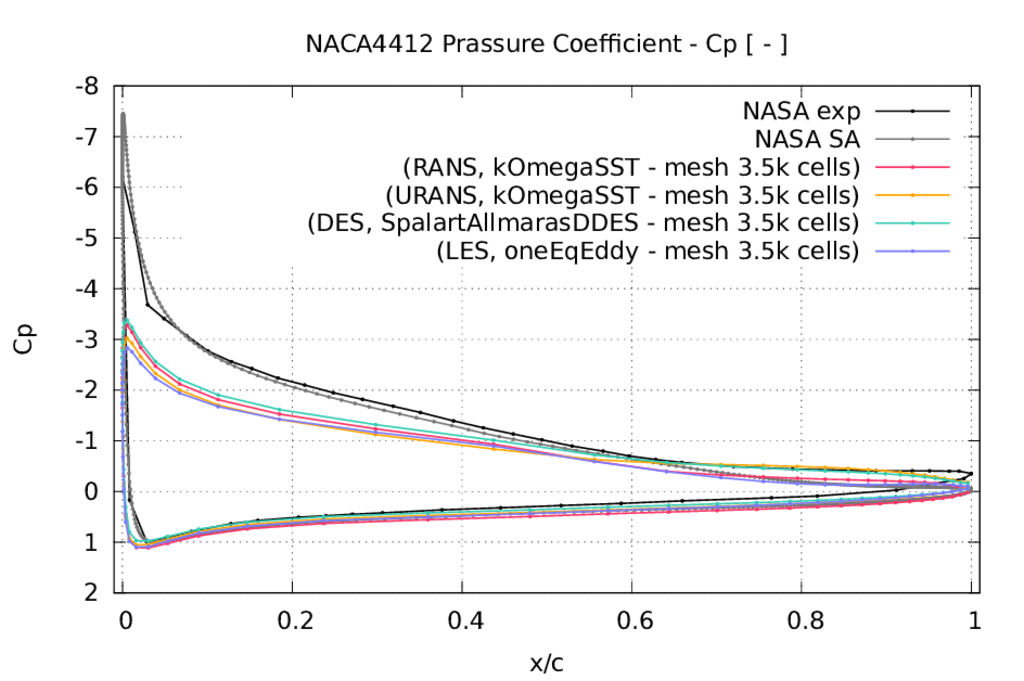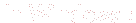Previous: RANS, , lowRe, Grid Up: NACA4412 Test Case Next: Appendix:         RANS Theory

This is an automatically generated documentation by LaTeX2HTML utility. In case of any issue, please, contact us at info@cfdsupport.com.

## RANS vs. URANS vs. DES vs. LES

The run script Allrun.sh settings for steady state RANS is following:

``` turbulence=RANS
solver=simpleFoam
mode=incompressible
wall=wallFunction
endTime=0.00005
deltaT=0.00000005
NProc=6
mesh=3.5k
turbulenceModel=kOmegaSST
```

The run script Allrun.sh settings for transient RANS is following:

``` turbulence=RANS
solver=pimpleFoam
mode=incompressible
wall=wallFunction
endTime=0.00005
deltaT=0.00000005
NProc=6
mesh=3.5k
turbulenceModel=kOmegaSST
```

The run script Allrun.sh settings for transient DES is following:

``` turbulence=LES
solver=pimpleFoam
mode=incompressible
wall=wallFunction
endTime=0.00005
deltaT=0.00000005
NProc=6
mesh=3.5k
LESModel=SpalartAllmarasDDES
```

The run script Allrun.sh settings for transient LES is following:

``` turbulence=LES
solver=pimpleFoam
mode=incompressible
wall=wallFunction
endTime=0.00005
deltaT=0.00000005
NProc=6
mesh=3.5k
LESModel=oneEqEddy```Figure: NACA4412: Comparison RANS vs. URANS vs. DES vs. LES turbulence modeling. Mesh 3.5k cells. Pressure coefficientversus dimensionless x-axis. The x-axis was divided by the length of the chord of the NACA4412 profile. Dataset NASA exp is the experimental data. Dataset NASA SA is the NASA RANS simulation results with Spalart Allmaras turbulence model.